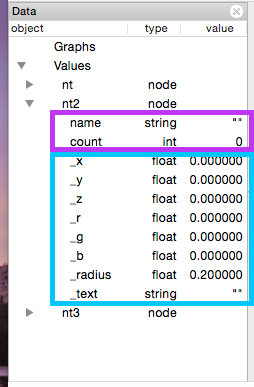# Graph Data

A graph is defined as a set of nodes and edges. Think of the nodes as the circles in the graph. The edges connect pairs of circles. In a social network, the nodes usually represent individuals. Links represent some type of "relationship" between pairs of individuals, usually friendship.

Continuing with the social graph example, what type of information might each node contain? Since the node represents an individual, let's call him "Bob", it can contain any information about that individual. Bob might have an age, height, birthdate, favorite color, and home address, just to name a few.

Defining graphs in GEL requires first defining a nodetype and linktype. Enter the following GEL commands into the GEL console (bottom right) to define different nodetypes.

node(string name) nt;             /* basic nodetype */
node(string name, int count) nt2; /* also contains node attribute count */
node(string id, int count=3) nt3; /* contains a default value for count */

node() nt;    /* will NOT work, must have at least ONE node attribute */


Notice how each one is shown in the Data panel. Expand each one to see the list of attributes.From the figure, the ones in purple are user defined attributes. The ones in blue, with an underscore prefix, are system defined attributes. The system defined attributes are the visual attributes for each node.

In the same way, you can define a linktype with its associated link attributes.

link[] lt;  /* undirected links */



Expand the linktypes in the data panel. You can also use the desc to display the contents of a node and link type in the console.

desc nt;
desc lt;


Visual attributes of nodes and links are denoted by an underscore prefix. These represent 3D coordinates (_x,_y,_z), color(_r,_g,_b), text and other visual attributes. The user should not define visual attributes as they are already included in the type definition.

node(string name,float _x) nt; //Will throw a syntax error


## Define a graph in GEL:

Graphs are defined within { }. Nodes are defined within ( ) and links are defined within [ ] or [ > depending on if links are undirected or directed. Defining a Graph in GEL with the following commands:

node(string name) nt;
graph(nt,lt)simple={("node1")[0.1,1]("node2")[0.2,2]("node3"), node1[0.3,3]node3};


Print the contents of the graph using desc (just the attribute names) or dump (all nodes and links) commands.

desc simple;   /* show node and link types */
dump simple;   /* show graph contents */


## Import a graph file

By default, the import command reads in a graph as a node list, a hyphen, and a link list. An example is shown below:

a
b
c
-
a,b,10.0
b,c,5.1
c,d,6.0


The Gel script to read in the above graph would be:

node(string id) nt;
graph(nt,lt)the40=import("filename.csv",",");


a,b,10.0
b,c,5.1
c,d,6.0


hello

node(string id) nt;
graph(nt,lt)the40=import("filename.csv",",",1); /* 1 means, no node list */


## Creating random graphs

graph(nt,lt) ran64=random(64);    //50% connectivity, default
graph(nt,lt) com64=random(64,100); //complete graph


Click on the ran64 and the40. You'll notice that both of them seem to only show one node. However, since by default, the position of all nodes are _x=0, _y=0, and _z=0, you will only see one node. Click on simple and type the following:

layout("cube");
dump simple;


Notice how the xyz coordinates are now different for each node. Try the following commands and watch the effect on the simple visualization:

foreach node in simple set_x=rand(-3,3),_y=rand(-3,3),_z=rand(-3,3);
foreach node in simple set_r=rand(),_g=rand(),_b=rand();
foreach node in simple set_r=rand(),_g=rand(),_b=rand();


## Saving graphs to be reloaded into Mango

save "state.txt"; /* save all graphs and variables */
clear;            /* clears all graph data */
run "state.txt";  /* reload saved graph data */

save "state.txt",simple; /* saves the simple graph instead of all graphs */


## Export graphs to be loaded into other programs

export("simplenew.tsv","tsv",simple); /* saves simple as a tab delimited file */
save "state.txt"; //saves all graphs as GEL commands


You can access specific attributes in a graph using the following:

node(string name) nt;
graph(nt,lt) g = {("node1")[1.0]("node2")};

g.node."node1"._x=10;


### Node Type

Since nodes are identified by unique strings, the basic node type is defined with one string attribute. Node is defined with the keyword node, list of attributes in parenthesis, and node type name. The list of attributes are a list of any of the primitive 4 data types (string, int, float, double).

node(string name) nt; /* most basic node type */
node(string id) nt;   /* can be named anything */


Nodes can have multiple attributes.

node(string name, int age, float weight, string birthdate) person;


Nodes can have default values

node(string name, int age=20, float weight=120, string birthdate="January 1, 1990") person;


You can combine node types:

node(string name, string color) nt;
node(string name, int age) nt2;
node(nt, nt2) nt3;

desc nt3; /* string name, string color, int age */


This allows combining graphs:

/* Graph 1 */
node(string id, int count) nt1;
graph(nt1,lt1) g1=random(10);

/* Graph 2 */
node(string id, string type) nt2;
graph(nt2,lt2) g2=random(10);

/* Combined Graph */
node(g1:node, g2:node) nt3;
graph(nt3,lt3) g3=g1;
g3.+=g2;


## Modifying a single node's attribute

Assigning a value to a single node's attribute has the following syntax.

/* create a basic 10 node random graph */
node(string id) nt;
graph(nt,lt) g=random(10);

/* layout randomly */
layout(g,"random");

/* change one node attribute */
g.node."n_1"._r=1;  /* change to red */
g.node."n_1"._text="hi!";
g.node."n_1"._text=g.node."n_1".id;